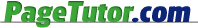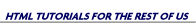What the hex is a hex code?

Let's learn a little about these "hex codes". In our everyday life we use a decimal (base 10) number system. We use the following numbers...

0-1-2-3-4-5-6-7-8-9

Somewhere along the line, computer people decided that certain things would be easier to do if they used a hexidecimal (base 16) number system...

0-1-2-3-4-5-6-7-8-9-A-B-C-D-E-F

Since we don't have a unique symbol for 10, 11, 12 etc, we use letters to represent those values. Here is a chart showing the hexidecimal equivalents from 0-255...

```  0  =  0    51  = 33    102 = 66    153 = 99    204 = CC
1  =  1    52  = 34    103 = 67    154 = 9A    205 = CD
2  =  2    53  = 35    104 = 68    155 = 9B    206 = CE
3  =  3    54  = 36    105 = 69    156 = 9C    207 = CF
4  =  4    55  = 37    106 = 6A    157 = 9D    208 = D0
5  =  5    56  = 38    107 = 6B    158 = 9E    209 = D1
6  =  6    57  = 39    108 = 6C    159 = 9F    210 = D2
7  =  7    58  = 3A    109 = 6D    160 = A0    211 = D3
8  =  8    59  = 3B    110 = 6E    161 = A1    212 = D4
9  =  9    60  = 3C    111 = 6F    162 = A2    213 = D5
10  =  A    61  = 3D    112 = 70    163 = A3    214 = D6
11  =  B    62  = 3E    113 = 71    164 = A4    215 = D7
12  =  C    63  = 3F    114 = 72    165 = A5    216 = D8
13  =  D    64  = 40    115 = 73    166 = A6    217 = D9
14  =  E    65  = 41    116 = 74    167 = A7    218 = DA
15  =  F    66  = 42    117 = 75    168 = A8    219 = DB
16  = 10    67  = 43    118 = 76    169 = A9    220 = DC
17  = 11    68  = 44    119 = 77    170 = AA    221 = DD
18  = 12    69  = 45    120 = 78    171 = AB    222 = DE
19  = 13    70  = 46    121 = 79    172 = AC    223 = DF
20  = 14    71  = 47    122 = 7A    173 = AD    224 = E0
21  = 15    72  = 48    123 = 7B    174 = AE    225 = E1
22  = 16    73  = 49    124 = 7C    175 = AF    226 = E2
23  = 17    74  = 4A    125 = 7D    176 = B0    227 = E3
24  = 18    75  = 4B    126 = 7E    177 = B1    228 = E4
25  = 19    76  = 4C    127 = 7F    178 = B2    229 = E5
26  = 1A    77  = 4D    128 = 80    179 = B3    230 = E6
27  = 1B    78  = 4E    129 = 81    180 = B4    231 = E7
28  = 1C    79  = 4F    130 = 82    181 = B5    232 = E8
29  = 1D    80  = 50    131 = 83    182 = B6    233 = E9
30  = 1E    81  = 51    132 = 84    183 = B7    234 = EA
31  = 1F    82  = 52    133 = 85    184 = B8    235 = EB
32  = 20    83  = 53    134 = 86    185 = B9    236 = EC
33  = 21    84  = 54    135 = 87    186 = BA    237 = ED
34  = 22    85  = 55    136 = 88    187 = BB    238 = EE
35  = 23    86  = 56    137 = 89    188 = BC    239 = EF
36  = 24    87  = 57    138 = 8A    189 = BD    240 = F0
37  = 25    88  = 58    139 = 8B    190 = BE    241 = F1
38  = 26    89  = 59    140 = 8C    191 = BF    242 = F2
39  = 27    90  = 5A    141 = 8D    192 = C0    243 = F3
40  = 28    91  = 5B    142 = 8E    193 = C1    244 = F4
41  = 29    92  = 5C    143 = 8F    194 = C2    245 = F5
42  = 2A    93  = 5D    144 = 90    195 = C3    246 = F6
43  = 2B    94  = 5E    145 = 91    196 = C4    247 = F7
44  = 2C    95  = 5F    146 = 92    197 = C5    248 = F8
45  = 2D    96  = 60    147 = 93    198 = C6    249 = F9
46  = 2E    97  = 61    148 = 94    199 = C7    250 = FA
47  = 2F    98  = 62    149 = 95    200 = C8    251 = FB
48  = 30    99  = 63    150 = 96    201 = C9    252 = FC
49  = 31    100 = 64    151 = 97    202 = CA    253 = FD
50  = 32    101 = 65    152 = 98    203 = CB    254 = FE
255 = FF
```

Below is a little widget that will convert any number back and forth from base 10 (our number system) to base 16 (hexidecimal). Type a decimal number in the left box and click the top button to convert to hexidecimal. Or, type a hex number in the right box and click the bottom button to convert it to decimal.

So when someone talks about a "hex code" or "hexadecimal number" or if you see cryptic color codes like 09EC0A, CCFFCC, 00AACC, etc., this is what they're talking about and where it comes from.

Taking it a little further, your computer understands colors in terms of red, green and blue values...

RED - GREEN - BLUE

or

R-G-B

Each of those can have a value from 0 to 255. 0 being the absence of color, 255 being full color. Using that scale, we get the following values...

 RED= 255-0-0 GREEN= 0-255-0 BLUE= 0-0-255 BLACK= 0-0-0 WHITE= 255-255-255

Since we have 3 colors, each with a value that can range from 0 to 255 we have 16,777,216 possible color combinations (256 ³). So, that's how we get 16.7 million colors. Black and white are not considered "colors". White is total saturation of all 3 colors and black is the total absence of any color (at least as far as your computer is concerned).

If we replace the decimal number with it's hexadecimal equivalent we start to get something that looks a little more like those "hex codes".

 RED= FF-0-0 GREEN= 0-FF-0 BLUE= 0-0-FF BLACK= 0-0-0 WHITE= FF-FF-FF

If we then use 00 for 0, and 01 for 1, etc., and if we get rid of the dashes(-), we then got ourselves some genuwine color codes!

 RED= FF0000 GREEN= 00FF00 BLUE= 0000FF BLACK= 000000 WHITE= FFFFFF

Here are a few random examples of hex color codes...

 200-0-0 C8-00-00 C80000 255-255-0 FF-FF-00 FFFF00 0-102-204 00-66-CC 0066CC 191-128-210 BF-80-D2 BF80D2 32-136-23 20-88-17 208817 231-156-3 E7-9C-03 E79C03 0-128-128 00-80-80 008080 71-64-7 47-40-07 474007

Notice when the red, green and blue values are equal, the result is a shade of gray...

 210-210-210 D2-D2-D2 D2D2D2 170-170-170 AA-AA-AA AAAAAA 117-117-117 75-75-75 757575 60-60-60 3C-3C-3C 3C3C3C

Well, I hope that helped you understand a little about "hex codes" and how they put color in your page. The more you understand how all this stuff works, the more control you'll have in your web page. You won't be limited to what some silly HTML editor will let you do. You can do just about anything you want.